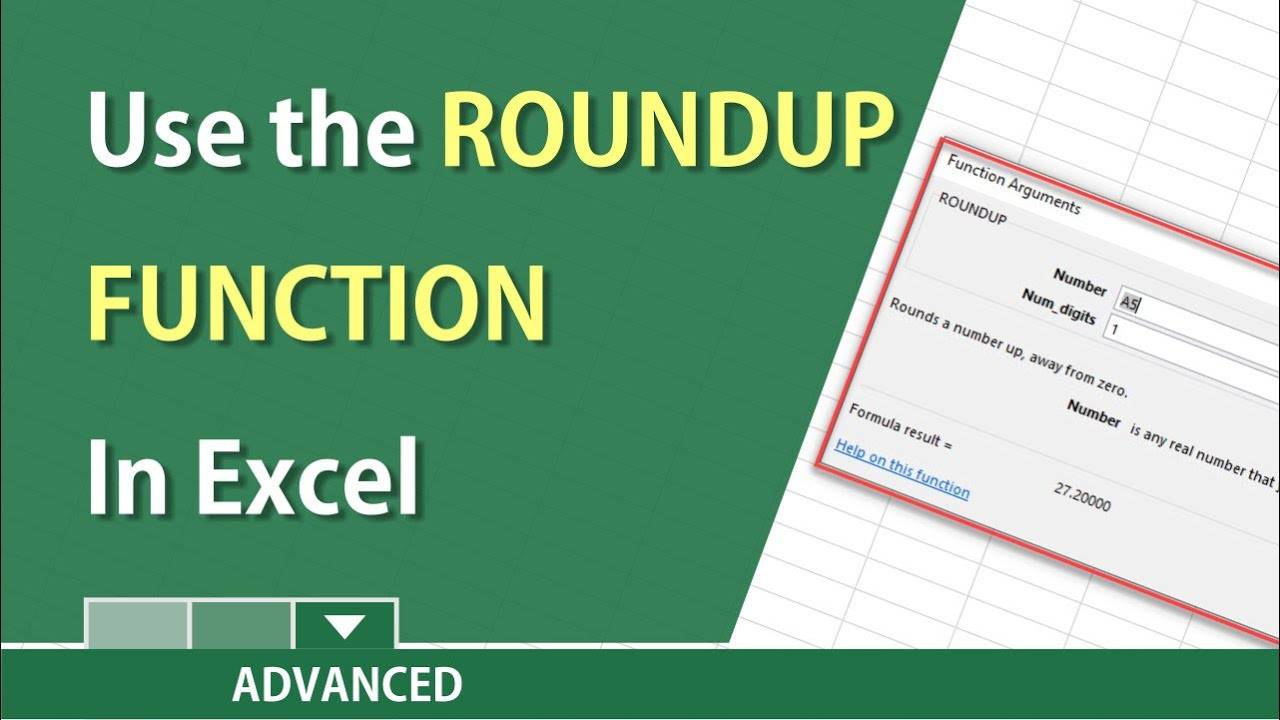# ROUNDUP Function in Excel by Chris MenardVideo on ROUND and MROUND in Excel

The ROUNDUP function is a great function. ROUNDUP always rounds number 1 to 9 up, compared to the ROUND function where numbers 5 and up are rounded up, and numbers 1 through 4 are rounded down.
The ROUNDUP function has two arguments, the cell reference and the number of decimals to round.

ROUNDUP Examples:
285.34 =roundup(cell,0) 286.00
285.34 =roundup(cell,1) 285.40
285.342 =roundup(cell,2) 285.35
285.342 =roundup(cell,-1) 290.00

Chris Menard’s Website:

Nguồn: https://rmacct.org/

Xem thêm bài viết khác: https://rmacct.org/cong-nghe/

• Chris, Can you have a formula that has =SUM() and then roundup that number in the same cell? Such as =SUM(E14)*F14+(E14) the answer will be 405 and then round up to the next 10 space so that it equals 410?Raw Gypsy Not Quite Hippie July 1, 2020 1:13 pm
• Great job. Very clear tutorial.Ryan de Medeiros July 1, 2020 1:13 pm